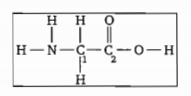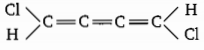The hybridisation of the  central  atom will change when:

(a) $N{H}_{3}$ combines with ${H}^{+}$

(b) ${H}_{3}B{O}_{{3}_{}}$combines with $O{H}^{-}$

(c) $N{H}_{3}$ forms $N{{H}_{2}}^{-}$

(d) combines with ${H}^{+}$

Concept Questions :-

Hybridisation
High Yielding Test Series + Question Bank - NEET 2020

Difficulty Level:

Spacies having maximum $\text{'}CI—O\text{'}$ bond order is:

Concept Questions :-

Polarity
High Yielding Test Series + Question Bank - NEET 2020

Difficulty Level:

Which of the following have $\mathrm{X}-\mathrm{O}-\mathrm{X}$ linkage?

(where X is Central atom)

(i) $C{r}_{2}{O}_{7}^{2-}$                            (ii) ${\mathrm{S}}_{2}{\mathrm{O}}_{3}^{2-}$

(iii) Pyrosilicate                     (iv) Hyponitrous Acid

(a) (i) (iii)                                             (b) (iii) (iv)

(c) (i) (iii) (iv)                                       (d) (i) (ii)

Concept Questions :-

Types of Bonding
High Yielding Test Series + Question Bank - NEET 2020

Difficulty Level:

Which bonds are formed by a carbon atom with $s{p}^{2}$ - hybridisation?

(a)

(b)

(c)

(d)

Concept Questions :-

Hybridisation
High Yielding Test Series + Question Bank - NEET 2020

Difficulty Level:

Which of the following species contains maximum number of atoms in 'XY' plane?

Concept Questions :-

Hybridisation
High Yielding Test Series + Question Bank - NEET 2020

Difficulty Level:

What are the hybridisation of the carbon atoms labelled ${C}_{1}$ and ${C}_{2}$ respectively in glycein?${C}_{1}$             ${C}_{2}$

(a) $s{p}^{2}$         (a) $s{p}^{2}$

(b) $s{p}^{2}$         (b) $s{p}^{3}$

(c) $s{p}^{3}$          (c) $s{p}^{2}$

(d) $sp$          (d) s${p}^{3}$

Concept Questions :-

Hybridisation
High Yielding Test Series + Question Bank - NEET 2020

Difficulty Level:

Choose the correct option for the following molecule :(a) non planar

(b) $\mu \ne 0$

(c) both (a) and (b)

(d) $\mu =0$

Concept Questions :-

Polarity
High Yielding Test Series + Question Bank - NEET 2020

Difficulty Level:

Select the correct statement:

(a)$HS{O}_{5}^{-}$ ion has one $\mathrm{S}—\mathrm{O}—\mathrm{H}$ linkage

(b) Number of $\mathrm{B}—\mathrm{O}—\mathrm{B}$ linkages in Borax is equal to number of $\mathrm{P}—\mathrm{O}—\mathrm{P}$ linkages in ${\mathrm{P}}_{4}{\mathrm{O}}_{10}$

(c) Hybridization of both sulphur on $\left({\mathrm{H}}_{2}{\mathrm{S}}_{2}{\mathrm{O}}_{5}\right)$ (pyrosulphurous acid) is name but oxidization state of both sulphur are different

(d) Tetra -Polyphosphoric has four $\mathrm{P}—\mathrm{O}—\mathrm{P}$ and no $\mathrm{P}—\mathrm{P}$ linkage

Concept Questions :-

Hybridisation
High Yielding Test Series + Question Bank - NEET 2020

Difficulty Level:

The H-O-H bond angles in ${H}_{3}{O}^{+}$ are approximately ${107}^{°}$. The orbitals used by oxygen in these bond are best describe as:

(a) $p-orbitals$

(b) sp-hybrid orbitals

(c) hybrid orbital

(d) $s{p}^{3}$. hybrid orbital

Concept Questions :-

Hybridisation
High Yielding Test Series + Question Bank - NEET 2020

Difficulty Level:

Which of the following molecular species is not linear?

(a) $\left(\mathrm{CN}{\right)}_{2}$                                                       (b) ${\mathrm{OCN}}^{-}$

(c) ${\mathrm{XeF}}_{2}$                                                         (d) ${\mathrm{S}}_{3}^{2-}$

Concept Questions :-

Hybridisation